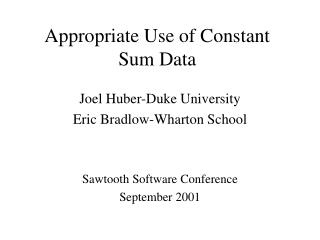DownloadDownload PresentationAppropriate Use of Constant Sum Data

# Appropriate Use of Constant Sum Data

Télécharger la présentation## Appropriate Use of Constant Sum Data

- - - - - - - - - - - - - - - - - - - - - - - - - - - E N D - - - - - - - - - - - - - - - - - - - - - - - - - - -
##### Presentation Transcript

1. Appropriate Use of Constant Sum Data Joel Huber-Duke University Eric Bradlow-Wharton School Sawtooth Software Conference September 2001

2. Appropriate Use of Constant Sum Data • What is Constant Sum Scale data? • When will CSS data work? • When will it fail? • An analysis of Volumetric Data using both HBsum and HBreg

4. Appropriate CSS usage • When people can estimate frequency of usage in a context—as examples: • Soft drink choice • Breakfast cereals • Prescriptions given diagnosis • Multiple supplier contracts

5. Inappropriate CSS usage • As a measure of preference strength • Allocate 10 points proportional to your preferences • As a measure of choice uncertainty • Indicate the probability of choosing each alternative • As a summary across different usage contexts • What proportion of beverage purchases will be Coke?

6. An example of conditional beverage choices • Drink Coke when tired • Drink Sprite when thirsty • Drink Heinekens with in-laws • Drink Iron City with friends • Drink Turning Leaf when romantic • Drink Ripple when depressed

7. Alternative to constant sum • Condition choices on usage situation • Derive situation frequency from a separate direct question • Ask a single choice questions • Derive variability by conditioning on context, or error in choice model

8. Analysis of Volumetric Choice Data • Volume estimates among four frequently purchased non-durables • Each alternative defined by brand, type, size, incentive and price • 10 different randomized sets of alternatives • One fixed holdout set • Task: How many of each would you choose? (max=10)

9. People reacted differently to this task • 22% of sets produced exactly one purchase • 33% of the sets produced none • 45% chose more than one purchase • People differed in their likelihood to use these strategies.

10. Two-stage analysis process • Need to model both choice share and volume • First stage: Constant sum model with ‘none’ option • Second stage: Hierarchical Bayes regression with item utilities from the first stage

11. Constant Sum Stage • Sawtooth’s HBSUM estimates 13 parameters for each person. • Model: Sums are normalized as if generated from five independent probabilistic choices • Choice weight =5 • Ten tasks equivalent to 50 independent probabilistic choices • None is included as a fifth alternative

12. Holdout choice accuracy • 78% hit rate • Mean average error predicting choice share 2.5 share points • Respondents differed strongly on their use of none

13. Heterogeneous response to None

14. Error predicting holdout share

15. HBreg predicts volume as a function of: • A constant for each individual • The utility of each item (from HBsum) • Adjusting for the utility of the set • Coefficient will be negative to the extent that volumes are proportional to the relative value within a set

16. Effectiveness of Dual Model • All coefficients significant and highly variable • Correlation between predicted and holdout volumes = .73

17. Error predicting holdout volumes

18. Conclusions • Constant sum scale measures are mainly appropriate when frequencies are easy to estimate given a set of alternatives • Volumetric estimates require even more of respondents, and thus are even more rare • Hierarchical Bayes methods are critical for correct modeling, because of the heterogeneity in the ways people respond to the task

19. Conclusions • We found heterogeneity with respect to • The use of None • The average volume • The partworths attached to the attributes • The degree to which alternatives are contrasted with others in the set • A two-stage HB allows people with idiosyncratic processes to be represented